# Meijer-G-functions

(diff) ← Older revision | Latest revision (diff) | Newer revision → (diff)

Generalizations of the hypergeometric functions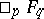of one variable (cf. also Hypergeometric function). They can be defined by an integral as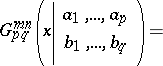where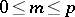,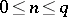and the parameters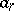,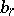are such that no pole of the functionscoincides with any pole of the functions. There are three possible choices for the contour:

a)goes from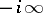toremaining to the right of the poles of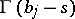and to the left of the poles of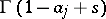;

b)begins and ends at, encircles counterclockwise all poles ofand does not encircle any pole of;

c)begins and ends at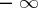, encircles clockwise all poles ofand does not encircle any pole of.

The integral converges if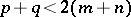,in case a); ifand eitheror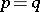andin case b); and ifand eitherorand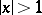in case c).

The integral defining the Meijer-functions can be calculated by means of the residue theorem and one obtains expressions for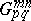in terms of the hypergeometric functions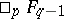or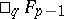. The functionsatisfies the linear differential equationwhereMany functions of hypergeometric type and their products can be expressed in terms of Meijer-functions, [a1]. For example,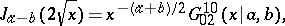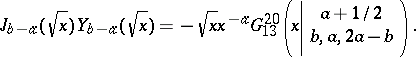Meijer-functions appear in the theory of Lie group representations (cf. also Representation of a compact group) as transition coefficients for different bases of carrier spaces of representations [a2].

How to Cite This Entry:
Meijer-G-functions. Encyclopedia of Mathematics. URL: http://encyclopediaofmath.org/index.php?title=Meijer-G-functions&oldid=13688
This article was adapted from an original article by A.U. Klimyk (originator), which appeared in Encyclopedia of Mathematics - ISBN 1402006098. See original article